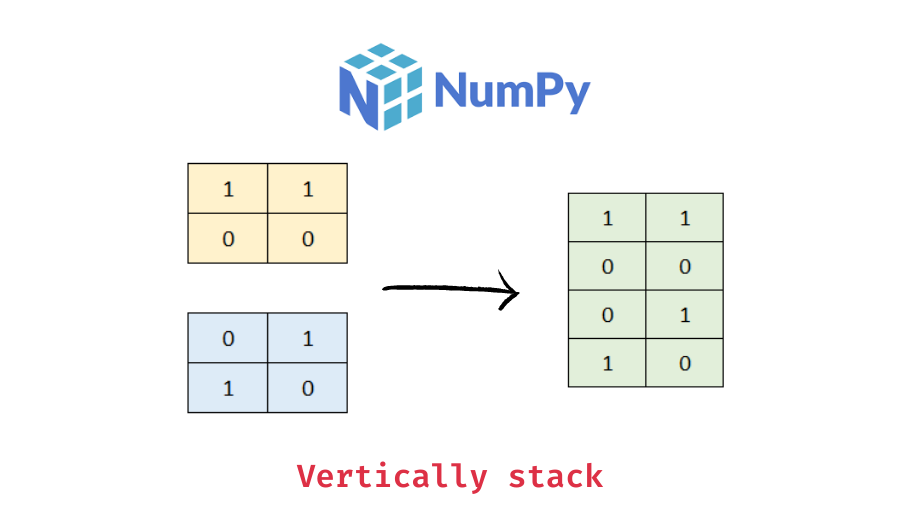# Using numpy vstack() to vertically stack arrays

In this tutorial, we will look at how to use the numpy vstack method to vertically stack (or concat) numpy arrays with the help of some examples.

You can use the numpy vstack() function to stack numpy arrays vertically. It concatenates the arrays in sequence vertically (row-wise). The following is the syntax.

```import numpy as np

# tup is a tuple of arrays to be concatenated, e.g. (ar1, ar2, ..)
ar_v = np.vstack(tup)```

It takes the sequence of arrays to be concatenated as a parameter and returns a numpy array resulting from stacking the given arrays.

Let’s look at some examples of how to use the numpy vstack() function.

Let’s stack two one-dimensional arrays together vertically.

```import numpy as np

# create two 1d arrays
ar1 = np.array([1, 2, 3, 4])
ar2 = np.array([5, 6, 7, 8])
# vstack the arrays
ar_v = np.vstack((ar1, ar2))
# display the concatenated array
print(ar_v)```

Output:

```[[1 2 3 4]
[5 6 7 8]]```

Here, we created two 1D arrays of length 4 and then vertically stacked them with the vstack() function. The resulting array is a 2D array of shape (2, 4).

You can also stack more than two arrays at once with the numpy vstack() function. Just pass the arrays to be stacked as a tuple. For example, let’s stack three 1D arrays vertically at once.

📚 Data Science Programs By Skill Level

Introductory

Intermediate ⭐⭐⭐

🔎 Find Data Science Programs 👨‍💻 111,889 already enrolled

Disclaimer: Data Science Parichay is reader supported. When you purchase a course through a link on this site, we may earn a small commission at no additional cost to you. Earned commissions help support this website and its team of writers.

```# create three 1d arrays
ar1 = np.array([1, 2, 3, 4])
ar2 = np.array([5, 6, 7, 8])
ar3 = np.array([1, 0, 0, 1])
# vstack the arrays
ar_v = np.vstack((ar1, ar2, ar3))
# display the concatenated array
print(ar_v)```

Output:

```[[1 2 3 4]
[5 6 7 8]
[1 0 0 1]]```

Here we concatenated three arrays vertically. The resulting array is of shape (3, 4). Similarly, you can stack multiple arrays, just pass them in the order you want as a sequence.

Now let’s stack a 1D array with a 2D array vertically.

```# create a 1d array
ar1 = np.array([1, 2, 3, 4])
# create a 2d array
ar2 = np.array([[0, 0, 0, 0],
[1, 1, 1, 1]])

# vstack the arrays
ar_v = np.vstack((ar1, ar2))
# display the concatenated array
print(ar_v)```

Output:

```[[1 2 3 4]
[0 0 0 0]
[1 1 1 1]]```

Here we vertically stacked a one-dimensional array of length 4 with a 2D array of shape (2, 4) resulting in a vertically stacked array of shape (3, 4).

You can also vertically stack two 2D arrays together in a similar way.

```# create two 2d arrays
ar1 = np.array([[1, 2, 3, 4],
[5, 6, 7, 8]])

ar2 = np.array([[0, 0, 0, 0],
[1, 1, 1, 1]])

# vstack the arrays
ar_v = np.vstack((ar1, ar2))
# display the concatenated array
print(ar_v)```

Output:

```[[1 2 3 4]
[5 6 7 8]
[0 0 0 0]
[1 1 1 1]]```

Here we stacked two 2d arrays of shape (2, 4) vertically resulting in an array of shape (4, 4).

For more on the numpy vstack() function, refer to its documentation.

With this, we come to the end of this tutorial. The code examples and results presented in this tutorial have been implemented in a Jupyter Notebook with a python (version 3.8.3) kernel having numpy version 1.18.5

•